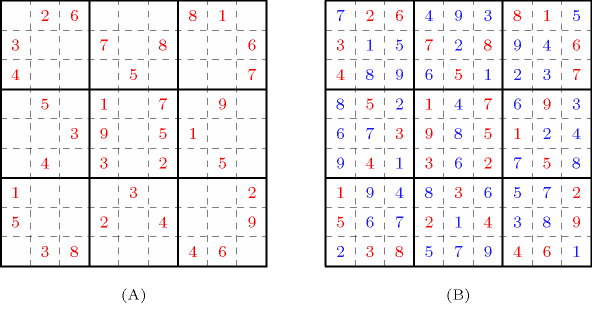### 3.7.250. Sudoku

A constraint that can be used for modelling the Sudoku puzzle problem. A Sudoku square is an $9×9$ array in which 9 distinct numbers in $\left[1,9\right]$ are arranged so that the following two conditions hold:

• Each number occurs once in each row and column.

• The numbers in each major $3×3$ block are distinct.

##### Figure 3.7.77. (A) A partially filled Sudoku square and (B) its unique completionThe Sudoku puzzle problem is to complete a partially filled board in order to get a Sudoku square. Part (A) of Figure 3.7.77 gives a partially filled Sudoku board, while part (B) provides its unique possible completion.